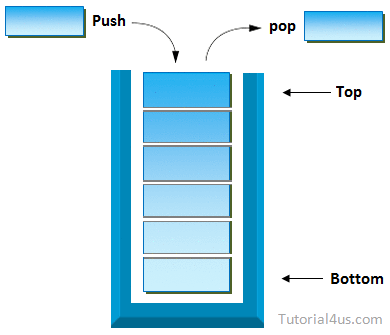# Stack in Java

## Stack in Collection Framework

Stack is one of the sub-class of Vector class so that all the methods of Vector are inherited into Stack.

The concept of Stack of Data Structure is implemented in java and develop a pre-defined class called Stack.## Stack Important Points

• Stack class allow to store Heterogeneous elements.
• Stack work on Last in First out (LIFO) manner.
• Stack allow to store duplicate values.
• Stack class is Synchronized.
• Initial 10 memory location is create whenever object of stack is created and it is re-sizable.
• Stack also organizes the data in the form of cells like Vector.
• Stack is one of the sub-class of Vector.

Creating a Stack is nothing but creating an object of Stsck Class.

## Syntax

```Stack s=new Stack();
```

### Constructors of Stack

• Stack(): is used for creating an object of Stack.

## Syntax

```Stack s=new Stack();
```

### Methods of Stack

• public boolean empty(): is used for returns true provided Stack is empty.It returns false in case of Stack is non-empty.
• public void push (Object): is used for inserting the elements into the Stack.
• public Object pop(): is used for removing Top Most elements from the Stack.
• public Object peek(): is used for retrieving Top Most element from the Stack.
• public int search(Object): is used for searching an element in the Stack.If the element is found then it returns Stack relative position of that element otherwise it returns -1, -1 indicates search is unsuccessful and element is not found.

## Example of Stack add Elements to Stack

```import java.util.*;
class StackDemo
{
public static void main(String args[])
{
Stack s=new Stack();

s.push(10);
s.push(20);
s.push(30);
s.push(40);
System.out.println("Stack data: "+s);//  [10,20,30,40]
}
}
```

## Output

```Stack data: [10,20,30,40]
```

## Example of Stack to add and remove Elements from Stack

```import java.util.*;
class StackDemo
{
public static void main(String args[])
{
Stack s=new Stack();

// Add the data to s
s.push(10);
s.push(20);
s.push(30);
s.push(40);
System.out.println("Stack elements: "+s);  //[10,20,30,40]

// Remove the top most element
System.out.println("Delete element: "+s.pop());  //40
System.out.println("Stack elements after pop: "+s);//  [10,20,30]
}
}
```

## Output

```Stack elements: [10,20,30,40]
Delete element: 40
Stack elements after pop: [10,20,30]
```

## Complete Example of Stack

```import java.util.*;
class StackDemo
{
public static void main(String args[])
{
Stack s=new Stack();
System.out.println("content of s="+s);    //[]
System.out.println("size of s="+s.size());   //10
System.out.println("Is empty?="s.empty());   //true

s.push(10);
s.push(20);
s.push(30);
s.push(40);
System.out.println("content of s="+s);  //[10,20,30,40]
System.out.println("size of s="+s.size());  //4
System.out.println("Is s empty ?=s.empty()");  //false
//remove the top most element
System.out.println("delete element="+s.pop());  //40
System.out.println("content of s after pop="+s);//  [10,20,30]
//extract the top most element
System.out.println("top most element="+s.peek());   //30
System.out.println("content of s after peek="+s);       //[10 20 30]
//Search the element 10 and 100
int srp=s.search(10);
System.out.println("stack relative pos.of 10 is="+srp);     //3
int srp1=s.search(100);
System.out.println("stack relative pos.of 100 is="+srp1);    //-1
}
}
```